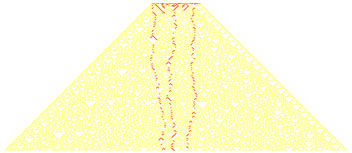#Wolfram Summer School

# Alumni# Ardeshir Kianercy

Summer School

Class of 2007

## Bio

After Ardeshir Kianercy received his BSc and MSc in mechanical engineering at Isfahan University of Technology in Iran, Ardeshir pursued his academic education in Canada at the University of Toronto. In 2004, he graduated with his second masters degree in chemical engineering from the University of Toronto, and was accepted at the University of Southern California in aerospace and mechanical engineering department where he is pursuing his PhD.

Ardeshir’s general interest is in the dynamics of the complex systems, and specifically dynamics of network evolution and the evolution of technology performance.

## Project: Network of Functional Rules: A Case Study of the Doubling Rules

One traditional engineering methodology in systems design is to reduce the behavior of the system and its elements to a simple mathematical model, and then to gradually improve the performance of the system by incrementally changing parameters. For example, one might find a differential equation that represents the output of an oscillatory circuit in terms of the values of several resistors and capacitors and then change the values of individual capacitors and resistors in turn in order to maximize the amplitude of the input signal.

I will investigate a model engineering problem based on the so-called ‘n to 2n‘ cellular automata. These CAs take a input as an initial condition of width n, and after a certain number of steps return a pattern of width 2n. As discussed in the NKS book on page 822, there are 4277 3-Cclor, range-one CA rules (found by exhaustive search) that solve the n to 2n problem for any n. Each of these rules solves the problem using a different detailed time evolution, and the number of steps required to find a solution varies considerably.

The purpose of this work is to take the 4277 known k=3, r=1 CA that solve the n to 2n problem and build a network that represents this solution space. One way to build such a network is to consider two CA rules (nodes) to be connected if their rule tables differ by one output (Hamming distance 1).The question then is, can one take a solution and find another one in an incremental fashion by, for example, changing one of the outputs in the rule table? How does one find optimal solutions (where more optimal solutions solve the problem in the least number of steps) using this incremental approach?

## Favorite Outer Totalistic Three-Color Rule

Rule chosen: 1697796This is rule 1697796 with initial condition {{1, 0, 2, 2, 2, 1, 2, 0, 1}, 0}.

• 21st Annual Wolfram Summer School
• Bentley University, Waltham, MA, USA
• June 25–July 15, 2023